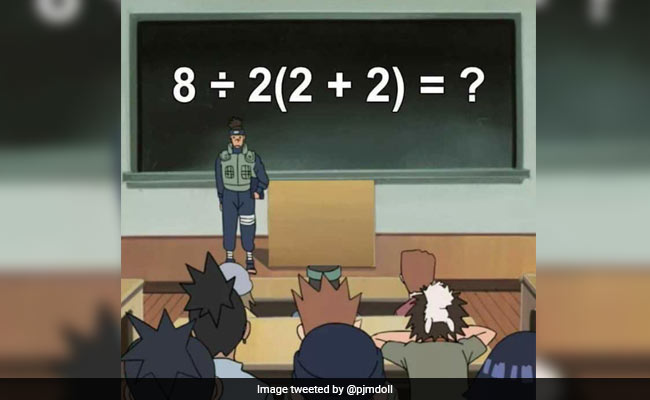Breaking News

# This ‘Simple’ Math Equation Has Divided The Internet. Can You Solve It?Can you solve this viral math equation?

At first glance it seems like school-level math. So why is this seemingly simple equation dividing the Internet? Posted by a Twitter user on Monday, 8 divided by 2(2+2) is the equation in question. Since being shared online, it has gone viral with over 12,000 ‘likes’ and thousands of comments.Can you solve this viral math equation?

At first glance it seems like school-level math. So why is this seemingly simple equation dividing the Internet? Posted by a Twitter user on Monday, 8 divided by 2(2+2) is the equation in question. Since being shared online, it has gone viral with over 12,000 ‘likes’ and thousands of comments. People can’t agree on the correct answer.

Can you solve it? Take a look:

Were you able to solve the equation? Did your answer come down to either 16 or 1?

Well, that’s where the trouble begins. Tweeple can’t seem to agree upon the correct answer.

So why is this happening? According to one Twitter user, the answer depends on which mathematician you listen to:

More simply put, the answer depends on whether you were taught the BODMAS or the PEMDAS method at school.

If you were taught the BODMAS method (Brackets, Orders, Division, Multiplication, Addition, Subtraction), you get 16 as the final answer.

On the other hand, if you used PEMDAS to solve the equation (Parentheses, Exponents, Multiplication, Division, Addition, Subtraction), then the answer is 1.